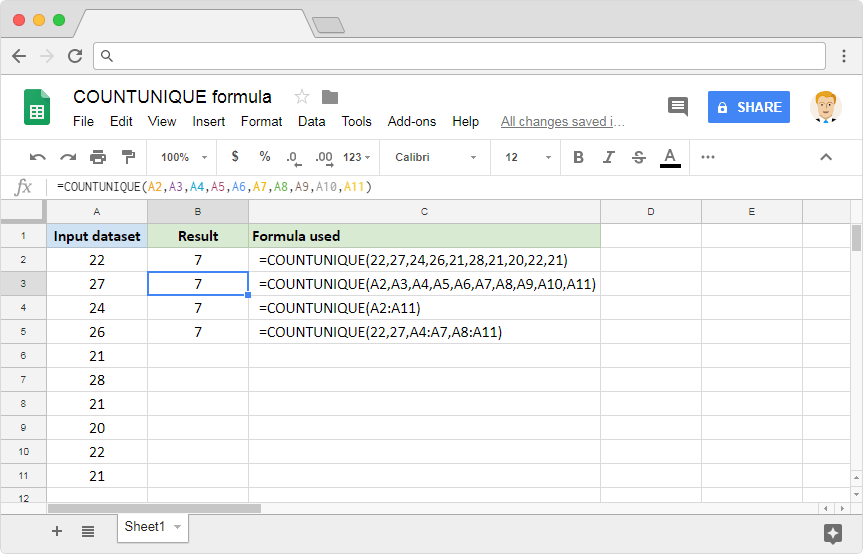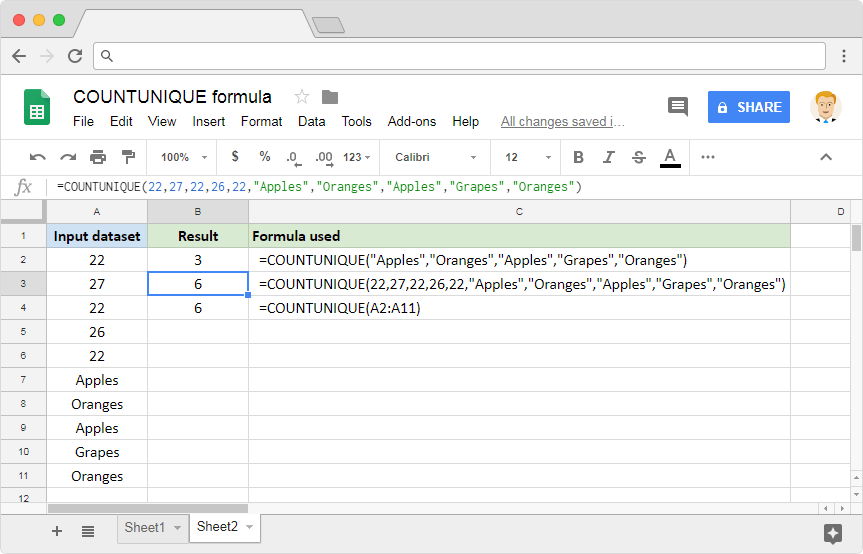# How to use the COUNTUNIQUE Google Sheets formulaWhile working with spreadsheets, we are bound to do many sorts of calculations. And we will, at times need to calculate unique occurrences of a dataset. The number of unique customers that shopped at a store, for example. How do we achieve this easily? We can, with the help of COUNTUNIQUE formula in Google Sheets application.

### Syntax

COUNTUNIQUE(value1, [value2, …])

• value1 – is the first input value in the dataset, which the formula has to identify the uniqueness from.
• value2, … – [ OPTIONAL ] – these are additional values in the dataset we are considering to identify the number of unique values from.

### Usage: COUNTUNIQUE formula in Google Sheets

We have just learned the syntax to the formula. Now it is time to dive headfirst into practically applying this newfound knowledge on the Google Sheets application. Take a look at the image below.The first example consumes direct numeric values and returns the count of unique instances of each value. The second example is a demonstration that shows us that the COUNTUNIQUE formula can use references to cells that store values. In the third example, instead of sending multiple values or references, we have passed a single composite range. This composite cell range consists of all the values from A2 through A11. And, in the final example, we not only passed direct numeric values but also a couple range references.

You’ll observe that the output is the same across all examples, regardless of the approach that we have taken with the formula. If we quickly validate the input dataset, we will indeed notice that there are 7 unique values within column A. And that is what the formula has returned.

#### Note

The COUNTUNIQUE formula is not just confined to numeric values. It can also consider strings for input values. We have demonstrated this capability in the following image.You can read more about the COUNT formula in the following blog: How to use the COUNT formula in Google Sheets.